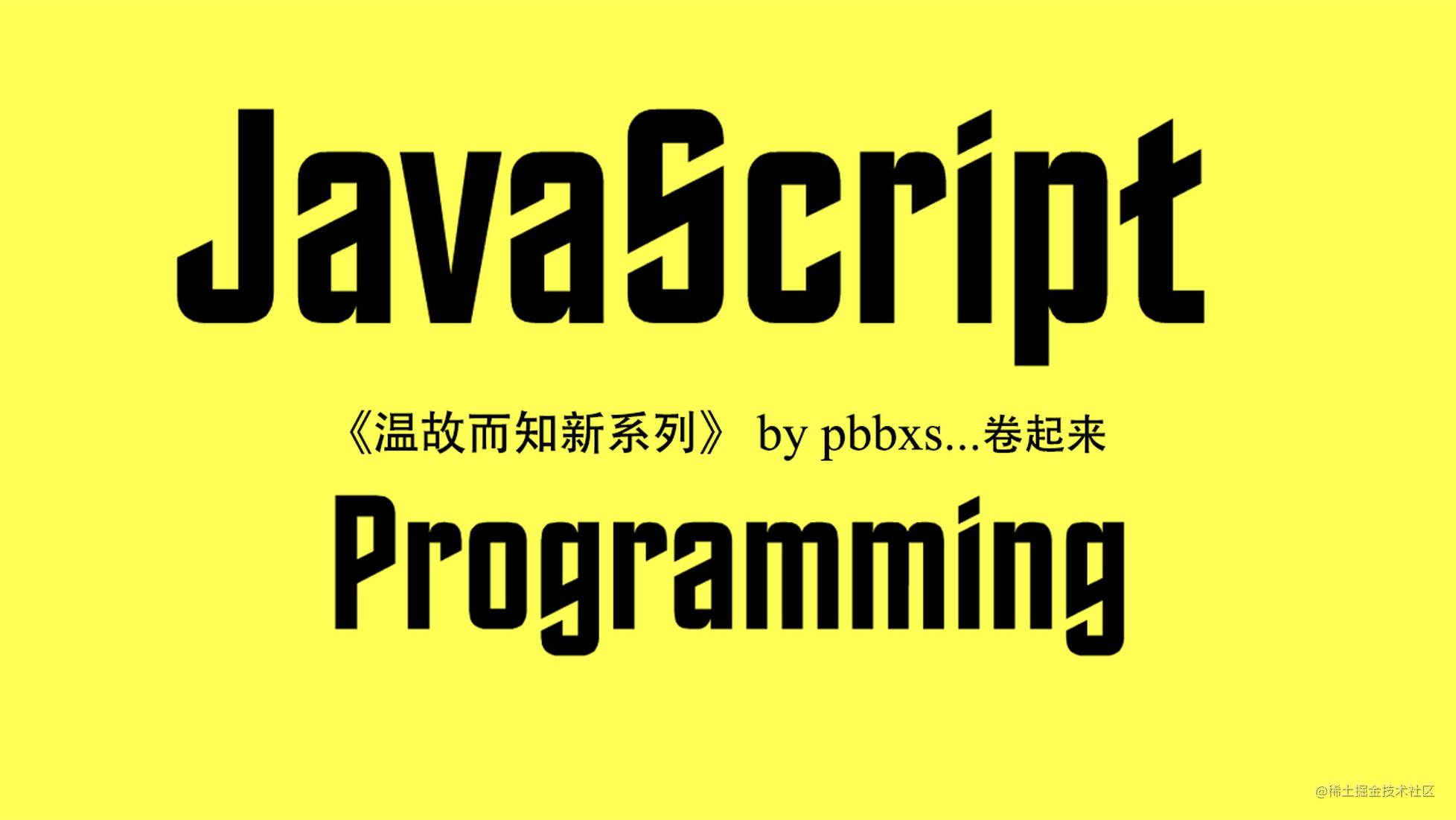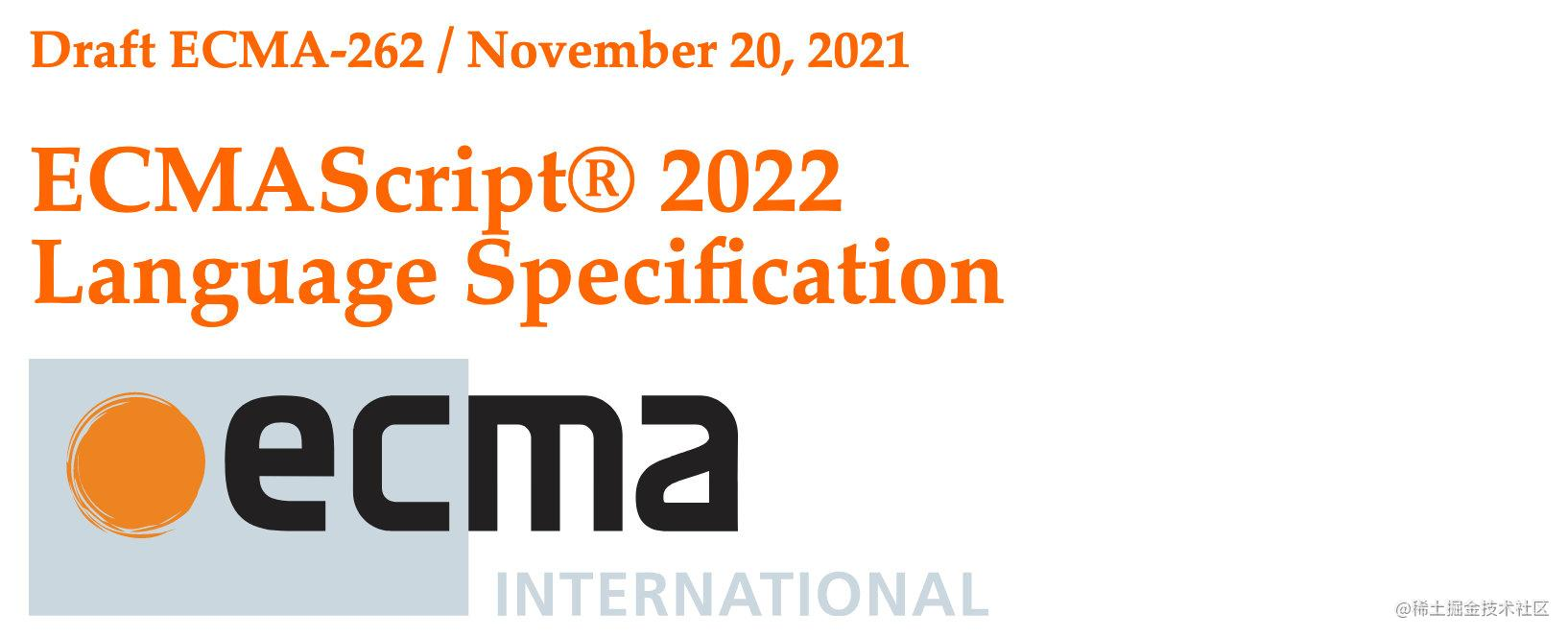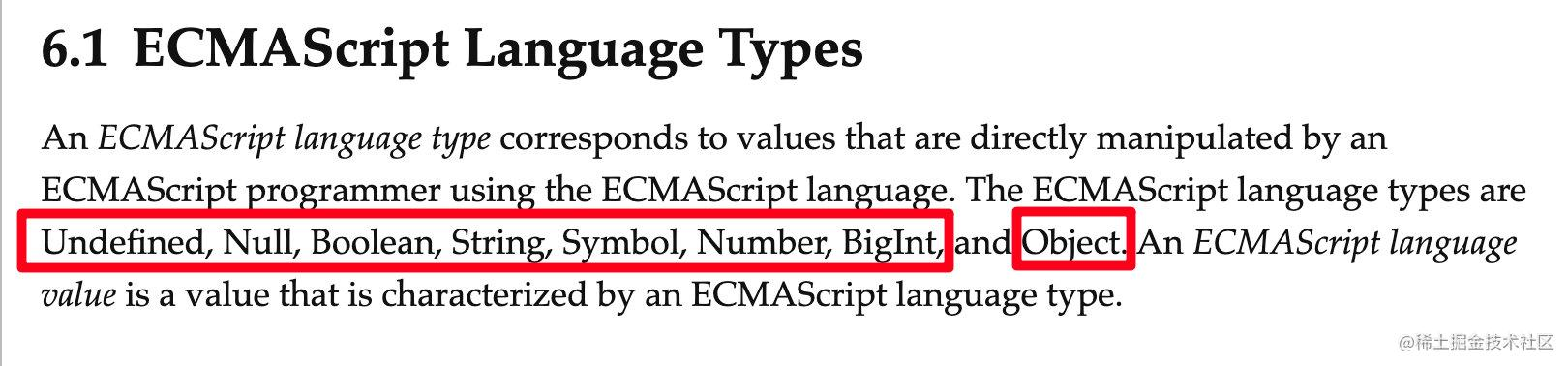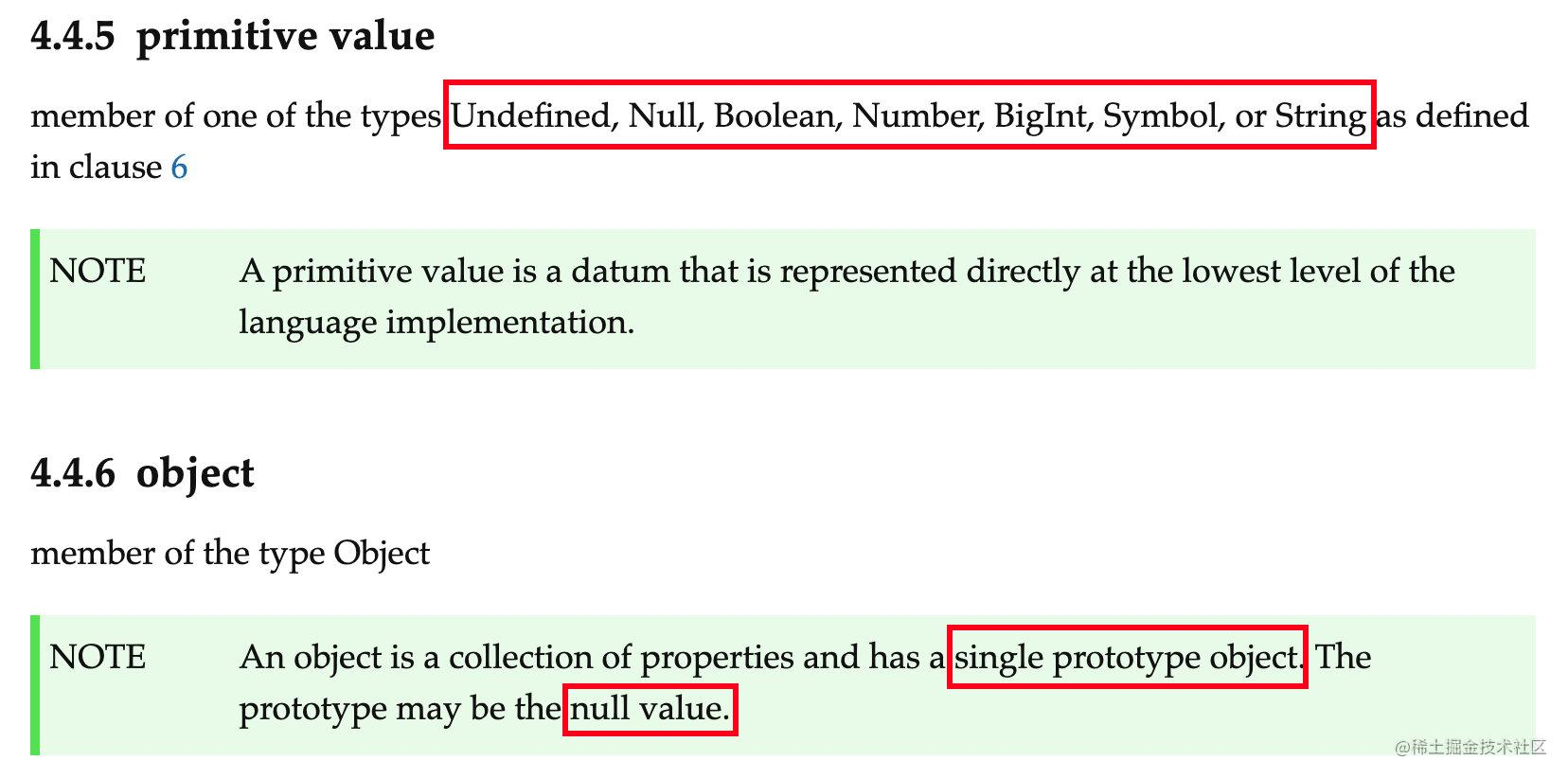# 温故而知新系列（一）你真的搞懂JavaScript数据类型了吗？快来缕缕吧！`JavaScript`是一门神奇的语言，就连数据类型这个入门科目都够你捣鼓半天## 一、数据类型分类`JavaScript`将数据类型分为`7`种基本数据类型`(Undefined,Null,Boolean,Number,String,Symbol,BigInt)``1`种复杂数据类型`(Object)`

• 基本数据类型存放的是原始值`primitive value`
• 复杂数据类型存放的是对象`an object`，即一个引用值`reference value`，其中有一个原型对象`prototype object`，可能为空（如顶级对象`Object``prototype object`即为空`{}`### 1. `typeof`操作符

`typeof`并不是一个函数，而是一个操作符，后面接一个操作数（一个表示对象或原始值的表达式），返回操作数的类型

``````//两种写法，返回值以字符串表示
typeof operand
typeof(operand)

Undefinedundefined(仅此一值)"undefined"
Nullnull(仅此一值)"object"
Booleantrue,false(仅此两值)"boolean"
Number-0,+0,1,2.1,Infinity,NaN..."number"
BigInt10n,20n..."bignit"
String"","jack","it is a joke"..."string"
SymbolSymbol("aaa")..."symbol"

Function对象const foo = function(){}"function"

### 2. Undefined

#### 2.1 声明未初始化

``````let a
console.log(a) //undefined
console.log(typeof a) //undefined

#### 2.2 未声明直接使用会报错

``````console.log(b) //ReferenceError: b is not defined
console.log(typeof b) //undefined

#### 2.3 `undefined`是一个假值

``````console.log(Boolean(undefined)) //false

### 3. Null

#### 3.1 表示空对象指针

``````console.log(typeof obj) //object

#### 3.2 `null`是一个假值

``````console.log(Boolean(null)) //false

#### 3.3 `undefined`是`null`的派生用法

``````console.log(undefined == null) //true

### 4. Boolean

#### 4.2 其他类型转布尔

`Boolean() `是转型函数，可以用于转换任意数据类型

``````//1.String
console.log(Boolean('')) //false
console.log(Boolean('li')) //true
//2.Number
console.log(Boolean(0)) //false
console.log(Boolean(NaN)) //false
console.log(Boolean(1)) //true
console.log(Boolean(Infinity)) //true
console.log(Boolean(-Infinity)) //true
//3.Object
console.log(Boolean({name: 'li'})) //true
//4.Undefined
console.log(Boolean(undefined)) //false
//5.Null
console.log(Boolean(null)) //false

### 5. Number

#### 5.1 如果数值本身是整数，小数点后面的0将被忽略

``````console.log(3.0) //3

#### 5.2 浮点数计算有精度丢失

``````console.log(0.1 + 0.2)  //0.30000000000000004

#### 5.3 值的范围

`ECMAScript `可以表示的最小数值保存在`Number.MIN_VALUE`中，这个值在多数浏览器中是 `5e-324`;可以表示的最大数值保存在 `Number.MAX_VALUE `中，这个值在多数浏览器中是` 1.797 693 134 862 315 7e+308`，如果某个计算的数值超出了`JavaScript`所能表示的范围，那么这个数值会被转换为正无穷`+Infinity`和负无穷`-Infinity`，这里我做了一个有意思的测试。

``````let max = Number.MAX_VALUE //1.7976931348623157e+308
let a = max + 1
console.log(isFinite(a)) //true
console.log(a) //1.7976931348623157e+308

``````let max = Number.MAX_VALUE //1.7976931348623157e308
let a291 = max + 1.7976931348623157e291
let b292 = max + 1.7976931348623157e292
console.log(isFinite(a291), isFinite(b292))  //true false
console.log(a291, b292) //1.7976931348623157e+308 Infinity

``````let min = Number.MIN_VALUE
let min1 = min - 5e-500
let min2 = min - 5e-325
console.log(isFinite(min1), isFinite(min2)) //true true
console.log(min1, min2) //5e-324 5e-324

#### 5.4 其他类型转数值

##### 5.4.1 Number()

`Number`是转型函数，可以用于转换任意数据类型

``````//null
console.log(Number(null)) //0
//undefined
console.log(Number(undefined)) //NaN

//Boolean
console.log(Number(true)) //1
console.log(Number(false)) //0

``````console.log(Number('')) //0
console.log(Number('a11')) //NaN
console.log(Number('011a11')) //NaN
console.log(Number('01.1a11')) //NaN
console.log(Number('011')) //11
console.log(Number('011.1')) //11.1
console.log(Number('0b11')) //3 二进制
console.log(Number('0o11')) //9 八进制
console.log(Number('0x11')) //17 16进制

``````//a是一个对象Boolean{false}，调用valueOf方法返回false
let a = new Boolean('')
//等价console.log(Number(a.valueOf())) <=>console.log(Number(false))
console.log(Number()) //0

let b = new Date()
console.log(Number(b))//得到的是当前时间的毫秒数

##### 5.4.2 parseInt

`parseInt()`函数更专注于字符串是否包含数值模式。字符串最前面的空格会被 忽略，从第一个非空格字符开始转换。如果第一个字符不是数值字符、加号或减号，`parseInt()`立即返回 `NaN`。这意味着空字符串也会返回`NaN`(这一点跟 `Number()`不一样，它返回` 0`)。如果第一个字符是数值字符、加号或减号，则继续依次检测每个字符，直到字符串末尾，或碰到非数值字符。`parseInt()`函数也能识别不同的整数格式(十进制、八 进制、十六进制)

``````console.log(parseInt('')) //NaN
console.log(parseInt('a1a11')) //NaN
console.log(parseInt('1a11')) //1
console.log(parseInt(' -11.1')) //-11
console.log(parseInt('0x13')) //19
console.log(parseInt('13', 16)) //16进制 19

##### 5.4.3 parseFloat

``````console.log(parseFloat(' a0.11a')) //NaN
console.log(parseFloat(' 01.1.1aa)) //1.1
console.log(parseFloat(' -0.1a')) //-0.1
console.log(parseFloat(' 0b11')) //0
console.log(parseFloat(' 02.0a')) //2

#### 5.5 NaN

``````console.log(0 / 0) //NaN
console.log(1 / 0) //Infinity
console.log(1 / -0) //-Infinity

• 任何涉及`NaN`的操作都返回`NaN`

• `NaN`不等于包括`NaN`在内的任何值

``````console.log(NaN == NaN) //false
复制代码``````
• `isNaN(param)`用于检验这个参数是否`不是数值`，把一个值传给`isNaN`后，该函数会尝试将它转换为数值，能转为数值则返回`false`，不能转换为数值则返回`true`

``````//能转换为数值的有 字符串数值如'10.1'或布尔值
//任何不能转换为数值的值都会导致该函数返回true
console.log(isNaN(true)) //1---false
console.log(isNaN(false)) //0---false
console.log(isNaN('10.1')) //10.1---false
console.log(isNaN('1')) //1---false
console.log(isNaN(NaN)) //true
console.log(isNaN('blue')) //true
console.log(isNaN(undefined)) //true
console.log(isNaN(null)) //0---false
复制代码``````

### 6. BigInt

`Number`中有最大最小安全整数，超出他们的范围的数值运算被认为是不安全的，为解决这个问题，就产生了`BigInt`，它一一种内置对象，`typeof`操作符返回`"bigint"`，并不是引用类型，而是存的原始值，使用在整数字面量后面加`n`的方式来表示，或者调用函数`BigInt()`，不可与`new`操作符配合使用，因为他构造出的不是对象实例，并不是引用类型

``````//BigIntConstructor. (value: bigint | boolean | number | string): bigint
let a = 1n
let b = BigInt(true)
console.log(typeof a) //bigint
console.log(a, b) //1n 1n

#### 6.1 操作符运算

``````console.log(1n + 1n) //2n
console.log(1n - 2n) //-1n
console.log(1n * 2n) //2n
console.log(5n / 2n) //2n 向下取整,带小数的部分会被舍弃
console.log(1n % 2n) //1n
console.log(3n ** 2n) //9n
console.log('程序员最喜欢的数字是: ', 1n << 10n) //程序员最喜欢的数字是:  1024n
console.log(10n >> 2n) //2n  分析:10n / (2n**2n) <=> 10n / 4n <=> 2n

#### 6.2 比较

##### 6.2.1 与Number相比较宽松相等，不严格相等
``````console.log(1n == 1) //true
console.log(1n === 1) //false

##### 6.2.2 可以和Number进行大小的比较
``````console.log(1n < 1) //false
console.log(2n >= 2) //true

#### 6.3 toString和valueOf

``````console.log(1n.toString()) //1
console.log(1n.valueOf()) //1n

### 7. String

#### 7.1 转义字符

`\`开头表示类似回车、换行等操作，与其他编程语言类似

`\xnn`表示以十六进制编码 `nn `表示的字符(其中 `n` 是十六进制数字` 0~F`)，例如`\x41` 等于`"A"`

`\unnnn`表示以十六进制编码 `nn `表示的`Unicode`字符(其中 `n` 是十六进制数字` 0~F`)，例如`\u03a3` 等于`"Σ"``emoji``Unicode `编码为`E63E``E757`

#### 7.2 其他类型转换为字符串

##### 7.2.1 String()转型函数

`null\undefined`分别返回`null\undefined`，其他类型参考下面的`toString`方法

``````console.log(String(null)) //null
console.log(String(undefined)) //undefined

##### 7.2.1 toString()方法

`null\undefined`没有该方法，数值、布尔值、对象和字符串都有该方法，数值、布尔值、和字符串在使用该方法是其实是自动转为了包装对象来使用的，后面会细说，对象直接调用其`toString`方法，有的重写了有的没有重写，没有重写的`toString`返回`[object type]`

``````let a = 1
console.log(a.toString()) //1
console.log(true.toString()) //true
console.log(false.toString()) //false
//对象
let obj1 = {
'': 'a' //键可以为空哦，不过一般不这么用
}
console.log(obj1.toString()) //[object Object]
let obj2 = {
'': 'obj2',
toString() {
return this['']
}
}
console.log(obj2.toString()) //[object Object]

``````let a = 10
console.log(a.toString()) //10
console.log(a.toString(2)) //1010
console.log(a.toString(8)) //12
console.log(a.toString(16)) //a

#### 7.3 模板字符串

ES6新增语法，使用**``**来表示字符串，内部将保留换行符，可以跨行定义字符串

``````let s = `
hello`
console.log(s.length) //6
console.log(s === '\n') //true
console.log(s === ' ') //true

##### 7.3.1 插值语法

``````let a = 1
let s = `the number is \${a}`
console.log(s) //the number is 1

##### 7.3.2 标签函数

``````function foo(x, y, z) {
console.log(x) //[ 'hello', 'world', '' ]
console.log(y) //second param
console.log(z) //third param
return 'foo function'
}
const res = foo`hello\${'second param'}world\${'third param'}`
console.log(res) //foo function

##### 7.3.3 原始字符串

``````function foo(str) {
console.log('实际显示的字符串为：')
for (const s of str) {
console.log(s)
}

console.log('原始值为：')
for (const s of str.raw) {
console.log(s)
}
}

//实际显示的字符串为：
// (这里是一个换行符)
//
// 原始值为：
// \u00a9
// \n

### 8. Symbol

``````let s1 = Symbol()
let s2 = Symbol('foo')
let s3 = Symbol('foo')
console.log(s1, s2, s3) //Symbol() Symbol(foo) Symbol(foo)
console.log(s2 === s3) //false
console.log(s2.description) //foo

#### 8.1 全局共享的Symbol

``````let s1 = Symbol.for('foo')
let des1 = Symbol.keyFor(s1)
console.log(des1) //foo
let s2 = Symbol.for('foo')
console.log(s1 === s2) //true

#### 8.2 属性描述符的3中使用方式

``````const s1 = Symbol('s1_Symbol')
const s2 = Symbol('s2_Symbol')
const s3 = Symbol('s3_Symbol')

/**
* 1. 定义字面量直接使用，使用计算属性
*/
const obj = {
name: 'dd',
age: 19,
[s1]: 'abc',
}

/**
* 2.属性名赋值，使用类似索引的赋值方式
*/
obj[s2] = 'bcd'

/**
* 3.使用Object.defineProperty
*/
Object.defineProperty(obj, s3, {
configurable: true,
enumerable: true,
writable: true,
value: 'cde',
})

console.log(obj)
/**
{
name: 'dd',
age: 19,
[Symbol(s1_Symbol)]: 'abc',
[Symbol(s2_Symbol)]: 'bcd',
[Symbol(s3_Symbol)]: 'cde'
}
*/

#### 8.3 遍历

``````console.log(Object.getOwnPropertySymbols(obj)) //此处的obj为8.2中的obj
//[ Symbol(s1_Symbol), Symbol(s2_Symbol), Symbol(s3_Symbol) ]

### 9. Object

• `constructor` 用于创建当前对象函数

• `hasOwnProperty(propertyName)` 用于判断当前对象实例(不是原型)上是否存在给定的属

• `isPrototypeOf(object)` 用于判断当前对象是否为另一个对象的原型

• `propertyIsEnumerable(propertyName)` 用于判断给定的属性是否可枚举，如属性的`enumerable``false`时，无法使用`in\for...in`进行遍历

• `toLocaleString()` 返回对象的字符串表示，该字符串反映对象所在的本地化执行环境

• `toString()`返回对象的字符串表示

• `valueOf()`返回对象对应的字符串、数值或布尔值表示，通常与 toString()的返回值相同

### 10. 包装类型（历史遗留问题）

``````let str1 = 'hello world.'
let str2 = str1.toUpperCase() //HELLO WORLD.
//等价于
let str1 = new String("hello world.") //创建一个String类型的实例
let str2 = str1.toUpperCase() //调用实例上的特定方法
str1 = null //销毁实例

#### 2.1 自动生命流程

``````let s1 = 'hello world.'
s1.info = 's1'
console.log(s1.info) //undefined

• 第2行代码创建了一个String实例，并在它身上加上了info属性，该行代码执行完毕该实例就会被销毁
• 第3行代码`又`创建了一个新的实例，并在它身上加上了info属性，但是并未进行赋值，该行代码执行完毕该实例就会被销毁

#### 2.2 Boolean、Number、String

`链接`JavaScript | MDN (mozilla.org)